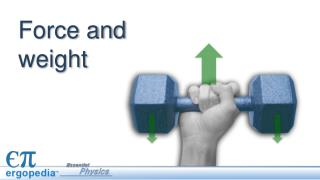Download PresentationForce and weight

# Force and weight - PowerPoint PPT PresentationDownload Presentation## Force and weight

- - - - - - - - - - - - - - - - - - - - - - - - - - - E N D - - - - - - - - - - - - - - - - - - - - - - - - - - -
##### Presentation Transcript

1. Force and weight

2. Explaining motion Isaac Newton was the first person to explain WHY objects move the way they do. He based his laws of motion on two key concepts: • mass • force

3. What is mass? All matter has mass and takes up space. A solid rock has mass. So do gases and liquids. With your hand out the window of a moving car, you feel the mass in the air pushing against you.

4. Measuring mass The SI unit for mass is the kilogram. This object has a mass of 2 kilograms. Kilogram video

5. What is force? The concept of force: What is force video.

6. What is force? The concept of force: A force is a push or a pull.

7. What is force? The concept of force: A force is a push or a pull. Forces can cause an object to change its motion. Can you give some examples of forces?

8. Types of forces The concept of force: A force is a push or a pull. Forces can cause an object to change its motion. Can you give some examples of forces? Examples of forces: • weight • friction • tension from rope • force from a spring • electric force

9. Units of force Quantitative: Force is measured in newtons.

10. Units of force

11. Weight is a type of force Weight is the force of gravity acting on objects with mass. How is weight different from mass? Weight vs Mass Video

12. Weight vs. mass Mass is an intrinsicproperty that measures the quantity of matter in an object. • Your mass does NOT change if you go into space.

13. Weight vs. mass Mass is an intrinsicproperty that measures the quantity of matter in an object. • Your mass does NOT change if you go into space. • Weight is an extrinsicproperty that depends on the gravity force. • Your weight changes if you go into space. Your weight depends on your location.

14. Weight vs. mass Mass is a scalar quantity. It has a magnitude (such as 60 kg), but no direction. Weight is a vector. It has magnitude AND direction. For objects on Earth, it always points straight down toward the center of the Earth. Also, known as the Force of gravity. Fg

15. Weight and mass Whenever you know the mass of an object, you can calculate its weight. Whenever you know the weight of an object, you can calculate its mass.

16. If you take a trip from Earth to the Moon, which statement below is correct? • Your mass and weight both decrease. • Your mass stays the same and your weight decreases. • Your mass decreases and your weight stays the same. • Your mass increases and your weight decreases. Assessment

17. If you take a trip from Earth to the Moon, which statement below is correct? • Your mass and weight both decrease. • Your mass stays the same and your weight decreases. • Your mass decreases and your weight stays the same. • Your mass increases and your weight decreases. Assessment

18. What is the weight of this dumbbell, in newtons? Assessment A 10 kg dumbbell is resting on a table partly supported by a spring that pulls upward with a force of 50 N.

19. What is the weight of this dumbbell, in newtons? Assessment Fg= mg = (10 kg)(9.8 m/s) = 98 N 98 N